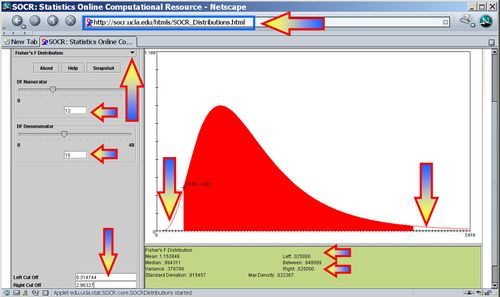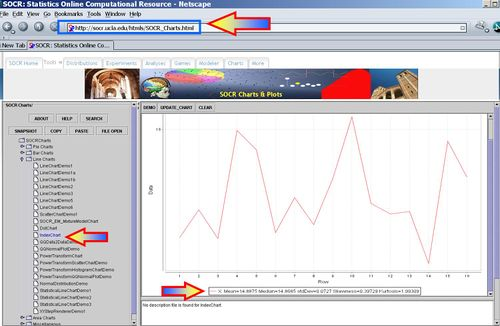# Difference between revisions of "AP Statistics Curriculum 2007 Infer BiVar"

## General Advance-Placement (AP) Statistics Curriculum - Comparing Two Variances

In the section on inference about the variance and the standard deviation we already learned how to do inference on either of these two population paparemters. Now we discuss the comparison of the variances (or standard deviations) using data randomly sampled from two different populations.

### Background

Recall that the sample-variance (s2) is an unbiased point estimate for the population variance $$\sigma^2$$, and similarly, the sample-standard-deviation (s) is a point estimate for the population-standard-deviation $$\sigma$$.

The sample-variance is roughly Chi-square distributed: $\chi_o^2 = {(n-1)s^2 \over \sigma^2} \sim \Chi_{(df=n-1)}^2$

### Comparing Two Variances ($$\sigma_1^2 = \sigma_2^2$$?)

Suppose we study two populations which are approximately Normally distributed, and we take a random sample from each population, {$$X_1, X_2, X_3, \cdots, X_n$$} and {$$Y_1, Y_2, Y_3, \cdots, Y_k$$}. Recall that $${(n-1) s_1^2 \over \sigma_1^2}$$ and $${(n-1) s_2^2 \over \sigma_2^2}$$ have $$\Chi^2_{(df=n - 1)}$$ and $$\Chi^2_{(df=k - 1)}$$ distributions. We are interested in assessing $$H_o: \sigma_1^2 = \sigma_2^2$$ vs. $$H_1: \sigma_1^2 \not= \sigma_2^2$$, where $$s_1$$ and $$\sigma_1$$, and $$s_2$$ and $$\sigma_2$$ and the sample and the population standard deviations for the two populations/samples, respectively.

Notice that the Chi-square distribution is not symmetric (it is positively skewed). You can visualize the Chi-Square distribution and compute all critical values either using the SOCR Chi-Square Distribution or using the SOCR Chi-square distribution calculator.

The Fisher's F distribution, and the corresponding F-test, is used to test if the variances of two populations are equal. Depending on the alternative hypothesis, we can use either a two-tailed test or a one-tailed test. The two-tailed version tests against an alternative that the standard deviations are not equal ($$H_1: \sigma_1^2 \not= \sigma_2^2$$). The one-tailed version only tests in one direction ($$H_1: \sigma_1^2 < \sigma_2^2$$ or $$H_1: \sigma_1^2 > \sigma_2^2$$). The choice is determined by the study design before any data is analyzed. For example, if a modification to an existent medical treatment is proposed, we may only be interested in knowing if the new treatment is more consistent and less variable than the established medical intervention.

• Test Statistic$F_o = {\sigma_1^2 \over \sigma_2^2}$

The higher the deviation of this ratio away from 1, the stronger the evidence for unequal population variances.

• Inference: Suppose we test at significance level $$\alpha=0.05$$. Then the hypothesis that the two standard deviations are equal is rejected if the test statistics is outside this interval

$H_1: \sigma_1^2 > \sigma_2^2$: If $$F_o > F(\alpha,df_1=n_1-1,df_2=n_2-1)$$

$H_1: \sigma_1^2 < \sigma_2^2$: If $$F_o < F(1-\alpha,df_1=n_1-1,df_2=n_2-1)$$

$H_1: \sigma_1^2 \not= \sigma_2^2$: If $$F_o < F(1-\alpha/2,df_1=n_1-1,df_2=n_2-1)$$ or $$F_o > F(\alpha/2,df_1=n_1-1,df_2=n_2-1)$$,

where $$F(\alpha,df_1=n_1-1,df_2=n_2-1)$$ is the critical value of the F distribution with degrees of freedom for the numerator and denominator, $$df_1=n_1-1,df_2=n_2-1$$, respectively.

In the image below the left and right critical regions are white with $$F(\alpha,df_1=n_1-1,df_2=n_2-1)$$ and $$F(1-\alpha,df_1=n_1-1,df_2=n_2-1)$$ representing the lower and upper, respectively, critical values. In this example of $$F(df_1=12, df_2=15)$$, the left and right critical values at $$\alpha/2=0.025$$ are $$F(\alpha/2=0.025,df_1=9,df_2=14)=0.314744$$ and $$F(1-\alpha/2=0.975,df_1=9,df_2=14)=2.96327$$, respectively.### Comparing Two Standard Deviations ($$\sigma_1 = \sigma_2$$?)

As the standard deviation is just the square root of the variance ($$\sigma = |\sqrt{\sigma^2}|$$), we do significance testing for the standard deviation analogously.

For Normally distributed random variables, given $$H_o: \sigma = \sigma_o$$ vs. $$H_1: \sigma \not= \sigma_o$$ , then $${(n-1) s^2 \over \sigma_o^2}$$ has a $$\Chi^2_{(df=n - 1)}$$ distribution, where $$s^2$$ is the square of the sample standard deviation.

### Hands-on activities

• Formulate appropriate hypotheses and assess the significance of the evidence to reject the null hypothesis for the population standard deviation ($$\sigma$$) assuming the observations below represent a random sample from the liquid content (in fluid ounces) of 16 beverage cans and can be considered as Normally distributed. Use a 90% level of confidence ($$\alpha=0.1$$).
 14.816 14.863 14.814 14.998 14.965 14.824 14.884 14.838 14.916 15.021 14.874 14.856 14.86 14.772 14.98 14.919
• Hypotheses$H_o: \sigma = 0.06 (\sigma_o)$ vs. $$H_1: \sigma \not= 0.06$$ .
• Get the sample statistics from SOCR Charts (e.g., Index Plot); Sample-Mean=14.8875; Sample-SD=0.072700298, Sample-Var=0.005285333.• Identify the degrees of freedom ($$df=n-1=15$$).
• Test Statistics$\Chi_o^2 = {(n-1)s^2 \over \sigma_o^2} \sim \Chi_{(df=n-1)}^2.$
• Significance Inference$\chi_o^2 = {15\times 0.005285333 \over 0.06^2}=22.022221$
P-value=$$P(\Chi_{(df=n-1)}^2 > \chi_o^2) = 0.107223$$. This p-value does not indicate strong evidence in the data to reject a possible population standard deviation of 0.06.
Error creating thumbnail: File missing

### More examples

• You randomly select and measure the contents of 15 bottles of cough syrup. The results (in fluid ounces) are shown. Formulate and test hypotheses about the standard deviation ($$\sigma_o=0.025$$) assuming the contents of these cough syrup bottles are Normally distributed. Is there data-driven evidence suggesting that the standard-deviation of the fluids in the bottles is not at an acceptable level?
 4.211 4.246 4.269 4.241 4.26 4.293 4.189 4.248 4.22 4.239 4.253 4.209 4.3 4.256 4.29
• The gray whale has the longest annual migration distance of any mammal. Gray whales leave Baja, California, and western Mexico in the spring, migrating to the Bering and Chukchi seas for the summer months. Tracking a sample of 50 whales for a year provided a sample mean migration distance of 11,064 miles with a standard deviation of 860 miles. Assume that the population of migration distances is Normally distributed to formulate and test hypotheses for the population standard deviation ($$\sigma_o=500$$).
• Use the hot-dogs dataset to formulate and test hypotheses about the population standard deviation of the sodium content in the poultry hot-dogs ($$\sigma_o=70$$).